# Tech Tips

### Characteristics of Double-Layer Capacitors

, Unlike a normal capacitor, a double-layer capacitor has a large electric capacity because the electric double-layer that is a layer with the opposite polarity to the electrode is formed around the electrode of the electrolyte. As with normal capacitors, it has very good high-current charge/discharge and repetitive cycle characteristics. Summarized briefly, it has the following features.

1. Able to charge and discharge several hundreds of thousands to several million cycles with less deterioration
2. High power density enables rapid charge and discharge
3. Environmental impact is low because lead and cadmium are not used for the electrodes
4. Like lead storage batteries, the usable temperature range is wide

Because of these features, a double-layer capacitor can be added between the inverter and the battery to enable high power input/output to the motor instead of the battery. Unlike normal capacitors, double-layer capacitors use electrolytes for their derivatives.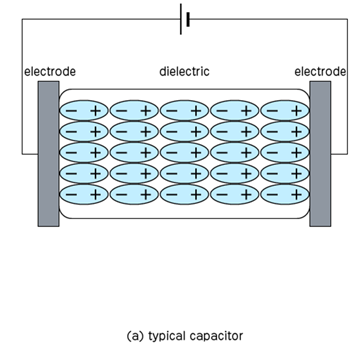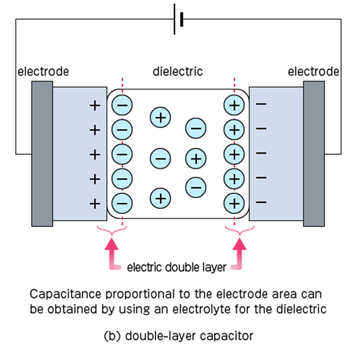Since cells of a typical double-layer capacitor are rated at around 2.5 to 2.7 V, it is necessary to connect the cells in series if high voltage is required. If capacity is required, connect in parallel.

However, when connecting in series, in order to prevent an overvoltage from being applied to individual cells by voltage imbalance, it is necessary to include a circuit for equalizing voltage-dividing resistors and voltages in parallel to each cell.

Recently, with the progress of activation technology for activated carbon, there is a new material with a very large specific surface area of about 2000 m2/g of activated carbon used for electrodes. This increases the capacity per weight in the double-layer capacitors. In addition, in cylindrical capacitors with excellent mass productivity, the electrical characteristics such as the capacity and resistance of internal elements change depending on the thickness and manufacturing method of the electrodes and the combination of activated carbon and electrolyte.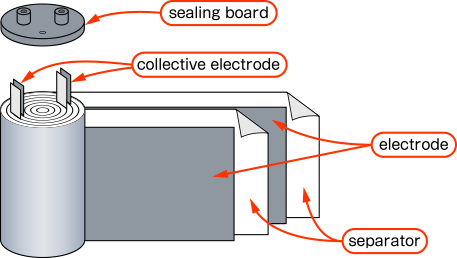Next, we explain the electrical characteristics of the double-layer capacitor. The first characteristic is for discharge. Unlike the storage battery, the voltage change is linear and can be discharged to 0 V, so it is easy to predict the remaining charge.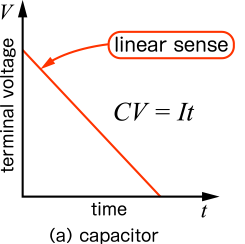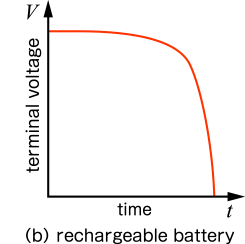However, there are four points that need to be considered that change with temperature: At first, it is "capacity." At very low temperatures, capacity tends to decrease. Likewise, the "DC internal resistance" increases. This is due to the combination of the resistivity of the constituent material and the internal connection resistance.

On the other hand, the "leakage current" and "voltage holding characteristics (self-discharge characteristics)" are affected when the temperature rises. At high temperatures, the leakage current increases, which also degrades the voltage holding characteristics.

### Application Example of Double-Layer Capacitor

We introduce the usage example of a double-layer capacitor such as "energy leveling," "energy regeneration," and "momentary voltage drop compensation device."

First, we introduce the usage example of "energy leveling." This is the usage when there is time fluctuation in the input energy. When there are many inputs, put a double-layer capacitor to keep the output lower than the input. If the input is low, the constant electricity is output by adding the electricity stored in the double-layer capacitor.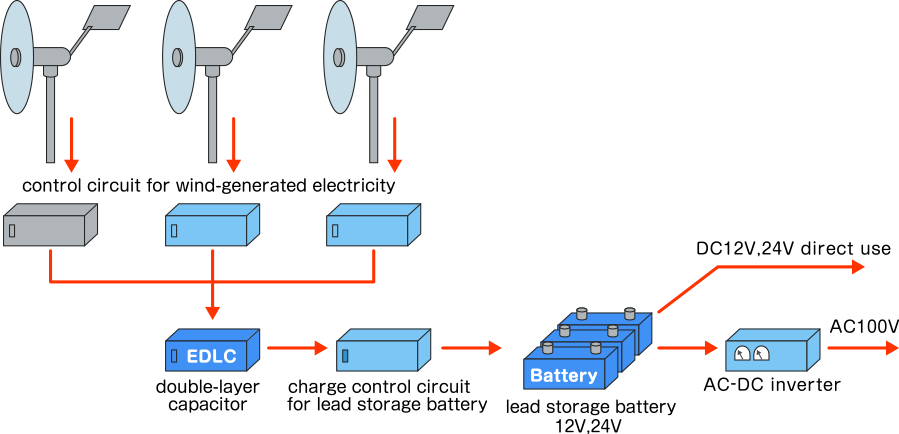For example, power plants that use green power such as wind power that is affected by the strength of the wind, and solar power that cannot generate power during the night, and that also depends on the number of clouds during the day, are targeted. Of course, the storage capacity of the double-layer capacitor is not so large, so the energy is actually stored in a NAS storage battery, redox flow storage battery, large lithium-ion battery, etc. In particular, because lithium-ion batteries use the CC/CV control as a charge control method for a full charge, an input with a certain amount of current is required. Therefore, things such as green energy that fluctuates in the amount of input current are undesirable on the input side. In some cases, a circuit is manufactured in which all generated electricity is temporarily stored in a double-layer capacitor and then output to a storage battery with constant output. However, some of them have begun to be used for power storage.

Second, about "energy regeneration". For example, in railways such as the Shinkansen, regenerative braking is used for braking to recover the electricity used for acceleration. This is another area where double-layer capacitors are suitable. It is also used by cranes, etc. By storing electricity in a double-layer capacitor at the time of hoisting the crane and using this energy at the time of hoisting, it is possible to reduce the fuel consumption of the engine and to make the energy more efficient.
In both cases, the energy that was previously discarded as frictional heat is recovered and used effectively.

Finally, we will introduce the "momentary voltage drop compensator." For example, when a power failure or momentary voltage drop occurs due to a lightning strike, this may cause the operation of the device to become unstable or even be destroyed in places where precision measuring equipment is used, such as factories or medical facilities. This measure can be taken by introducing a device using a double-layer capacitor. If it is likely to go out of power for a long time, it is possible to compensate until it switches to a private electric generator, and it is possible to safely afford the time to shut down the device.

Thus, double-layer capacitors are used in various places as a buffer to stabilize the input current.